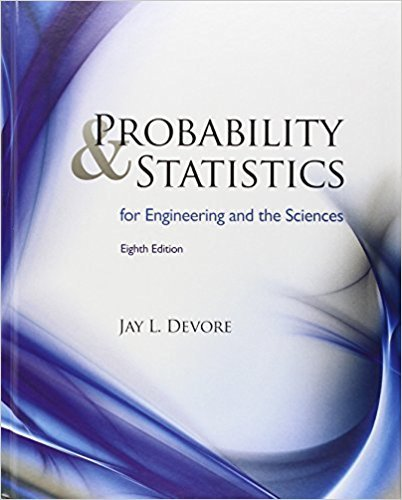×
×

# Solutions for Chapter 2: Probability## Full solutions for Probability and Statistics for Engineering and the Sciences | 8th Edition

ISBN: 9780538733526Solutions for Chapter 2: Probability

Solutions for Chapter 2
4 5 0 430 Reviews
15
2
##### ISBN: 9780538733526

Probability and Statistics for Engineering and the Sciences was written by and is associated to the ISBN: 9780538733526. This textbook survival guide was created for the textbook: Probability and Statistics for Engineering and the Sciences , edition: 8. Chapter 2: Probability includes 114 full step-by-step solutions. Since 114 problems in chapter 2: Probability have been answered, more than 25069 students have viewed full step-by-step solutions from this chapter. This expansive textbook survival guide covers the following chapters and their solutions.

Key Statistics Terms and definitions covered in this textbook
• Asymptotic relative eficiency (ARE)

Used to compare hypothesis tests. The ARE of one test relative to another is the limiting ratio of the sample sizes necessary to obtain identical error probabilities for the two procedures.

• Average run length, or ARL

The average number of samples taken in a process monitoring or inspection scheme until the scheme signals that the process is operating at a level different from the level in which it began.

• Axioms of probability

A set of rules that probabilities deined on a sample space must follow. See Probability

• Biased estimator

Unbiased estimator.

• Bivariate normal distribution

The joint distribution of two normal random variables

• Box plot (or box and whisker plot)

A graphical display of data in which the box contains the middle 50% of the data (the interquartile range) with the median dividing it, and the whiskers extend to the smallest and largest values (or some deined lower and upper limits).

• Cause-and-effect diagram

A chart used to organize the various potential causes of a problem. Also called a ishbone diagram.

• Chance cause

The portion of the variability in a set of observations that is due to only random forces and which cannot be traced to speciic sources, such as operators, materials, or equipment. Also called a common cause.

• Chi-square test

Any test of signiicance based on the chi-square distribution. The most common chi-square tests are (1) testing hypotheses about the variance or standard deviation of a normal distribution and (2) testing goodness of it of a theoretical distribution to sample data

• Components of variance

The individual components of the total variance that are attributable to speciic sources. This usually refers to the individual variance components arising from a random or mixed model analysis of variance.

• Confounding

When a factorial experiment is run in blocks and the blocks are too small to contain a complete replicate of the experiment, one can run a fraction of the replicate in each block, but this results in losing information on some effects. These effects are linked with or confounded with the blocks. In general, when two factors are varied such that their individual effects cannot be determined separately, their effects are said to be confounded.

• Control chart

A graphical display used to monitor a process. It usually consists of a horizontal center line corresponding to the in-control value of the parameter that is being monitored and lower and upper control limits. The control limits are determined by statistical criteria and are not arbitrary, nor are they related to speciication limits. If sample points fall within the control limits, the process is said to be in-control, or free from assignable causes. Points beyond the control limits indicate an out-of-control process; that is, assignable causes are likely present. This signals the need to ind and remove the assignable causes.

• Control limits

See Control chart.

• Crossed factors

Another name for factors that are arranged in a factorial experiment.

• Curvilinear regression

An expression sometimes used for nonlinear regression models or polynomial regression models.

• Discrete uniform random variable

A discrete random variable with a inite range and constant probability mass function.

• Error of estimation

The difference between an estimated value and the true value.

• Error variance

The variance of an error term or component in a model.

• Gamma random variable

A random variable that generalizes an Erlang random variable to noninteger values of the parameter r

• Generator

Effects in a fractional factorial experiment that are used to construct the experimental tests used in the experiment. The generators also deine the aliases.

×